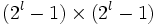Central and additive group of a commutative unital ring implies potentially iterated commutator subgroup in solvable group

(diff) ← Older revision | Latest revision (diff) | Newer revision → (diff)

Statement

Suppose$G$ is a solvable group and$H$ is a central subgroup that is isomorphic to the additive group of a commutative unital ring. Then,$H$ is a Potentially iterated commutator subgroup (?) of$G$, i.e., there exists a group$K$ containing$G$ such that$H$ is an iterated commutator subgroup of$K$.

In particular,$H$ is a Potentially verbal subgroup (?) and a Potentially fully invariant subgroup (?) of$G$.

Proof

Given: A solvable group$G$, a central subgroup$H$ of$G$, a commutative unital ring$R$ such that$H$ is isomorphic to the additive group of$R$.

To prove: There exists a group$K$ containing$G$ such that$H$ is an iterated commutator subgroup of$K$.

Proof: Suppose$G$ has derived length$l$. Consider$L = U(2^{l+1} - 1,R)$, i.e., the group of$(2^l - 1) \times (2^l - 1)$ upper-triangular matrices with$1$s in the diagonal and entries from$R$, under multiplication.$L$ has derived length$l + 1$ and its center as well as the$l^{th}$ member of its derived series is a subgroup isomorphic to$H$. Let$K$ be the central product of$G$ and$L$ with the center of$L$ identified with$H$.

Then, the$l^{th}$ member of the derived series of$K$ equals the common subgroup$H$. Thus,$H$ is an iterated commutator subgroup of$K$.